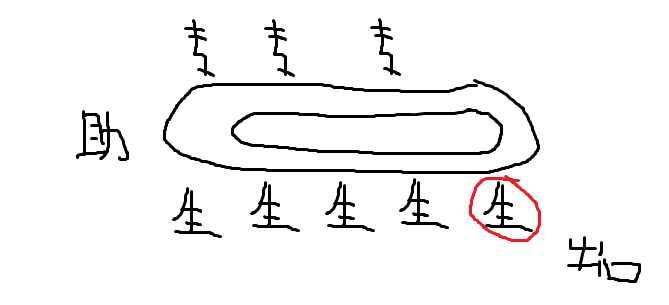# 国科大1. 自我介绍，2分钟/人（顺序54321）
2. 「你高中做过的印象最深的实验是什么」（顺序12345）
3. 「关于计算机科学与技术和人工智能，你有什么了解？你能否谈谈你对元宇宙的认识？」（顺序32415）
4. 「数学中复数的发现有什么意义，你怎么认识？」（顺序忘了）
5. 「你在疫情期间遇到了什么挫折，你怎么应对的？」（顺序忘了）

# 南大

1. (15pts) 证明：x^2+y^2+z^2-4x=-3,xy+2yz+xz-3x^2+x=4无实数解

1. (15pts) 从1,2,...n中随机选出一个数X，再从1,2,...,X中随机选出一个数Y，求Y期望。

2. (15pts)在\triangle ABC中，AB=7,AC=8,BC=9，I为内心，内接圆切BC,AC,AB于D,E,F。做DP垂直于EF于P。求IP。

3. (15pts)x^2-y!=2023的所有正整数解？

4. (20pts)p质数，已知1<a<b<p。若p在a进制下表示为\sum a_ia^i,若p在b进制下表示为\sum b_ib^i，令f(x)=\sum a_ix^i,g(x)=\sum b_ix^i。证明存在c>b,g(c)不是f(c)因数

5. (20pts) （不确定）q=2^{2^n}+3^{2^n},m=2^{n+1},证明m!\prod_{k=1}^{m-1}(q^k-1)因数

THE END